Lecture 24: Consensus 2

Reminder

Final Project: Short video due next Wednesday

• Not more than 10 minutes
• Simple presentation, audience = classmates
1. Describe problem you solved
2. Overview of solution method (sequential)
3. Opportunities for parallelism
4. Challenges and/or successes
• Don’t need final results!
• Simplest method: record in Zoom

Last Time

Introduced the consensus problem:

• $n$ processes, each with private input
• some processes may crash
• must produce output satisfying following properties
• Agreement: all processes output the same value
• Validity: if all systems have the same input, they all output that value
• Termination: all (non-faulty) processes decide on an output and terminate after a finite number of steps

Our Goal

Theorem (FLP, 1985). There is no algorithm that achieves consensus in the presence of even a single faulty process.

• Special case: there is no wait-free protocol for consensus for any $n > 1$
• wait-free is stronger assumption than termination
• Consider binary consensus all inputs 0/1

1. Model
2. Bivalent executions
• executions that can be extended to produce output 0 or 1
3. Critical executions
• if any processor takes a step, then output is determined
4. Proof of FLP result

Executions

An execution $E$ of algorithm $A$ specifies

• Inputs of all processes
• Sequence of steps taken by processes
• write
• terminate
• crash

Executions may be incomplete

• Not all nodes have terminated/crashed yet
• encodes current state/history of execution

Executions may be extended by scheduling more steps

Example Algorithm

Default to 0: output 0 unless all processes have input 1

int in = getLocalInput();

write(i, in); // write my value to register i

if (in == 0) return 0;

for (int j = 0; j < nProcesses; j++) {
// wait until register j has been written

if (read(j) == 0) return 0;
}

// all processors have in == 1
return 1;

Example of Execution $E$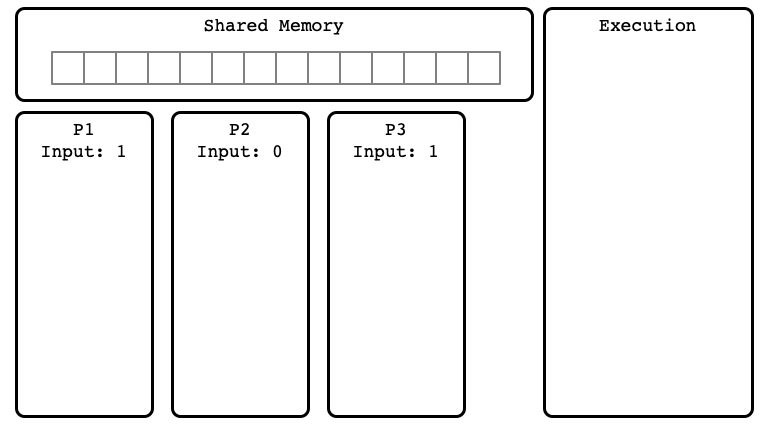$E$ Step 01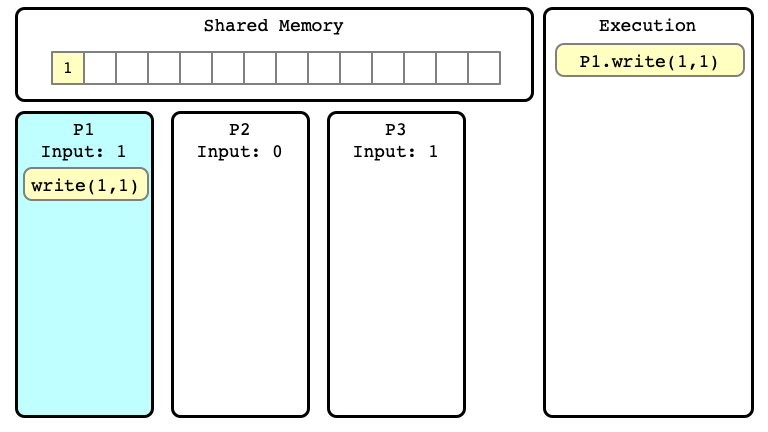$E$ Step 02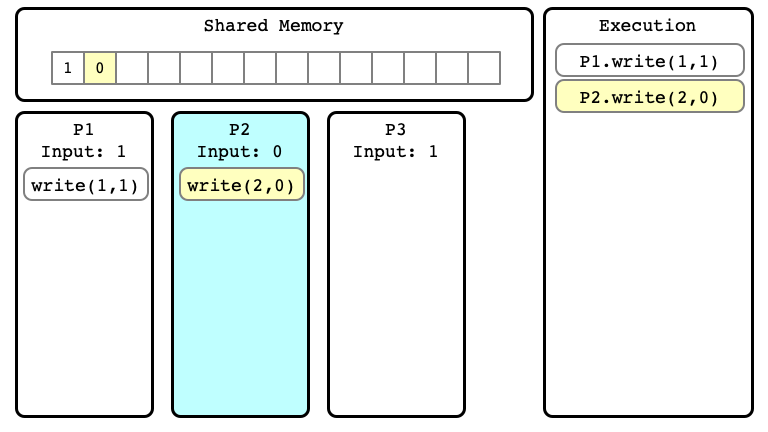$E$ Step 03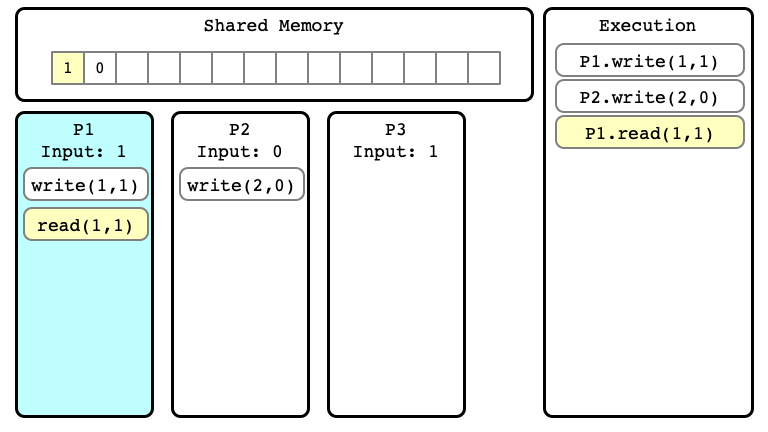$E$ Step 04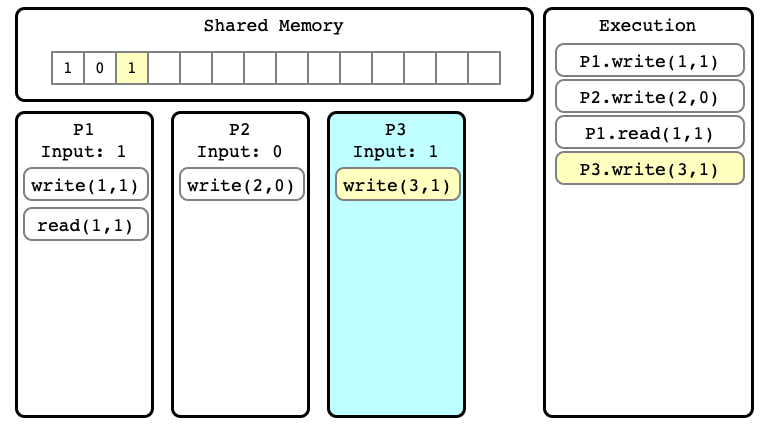$E$ Step 05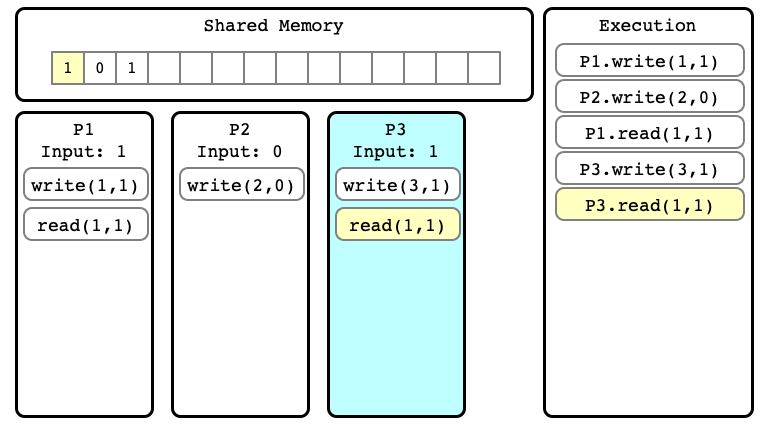$E$ Step 06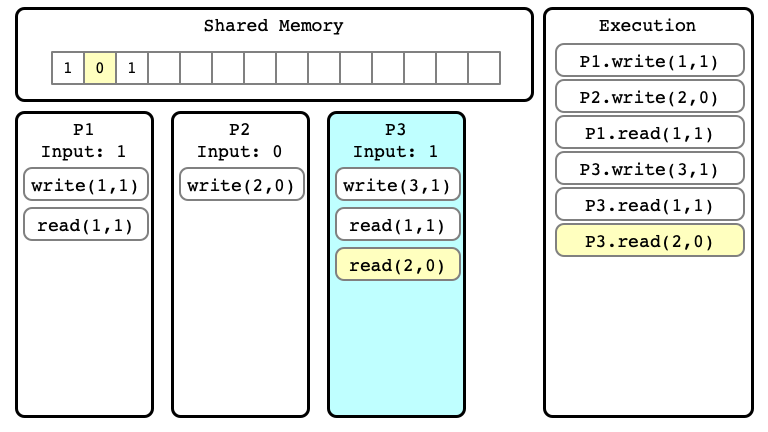Extending Executions

In $E$, no process has terminated yet

• We can consider extensions of a given execution
• Start with $E$, and perform more steps

$E’$ Step 06$E’$ Step 07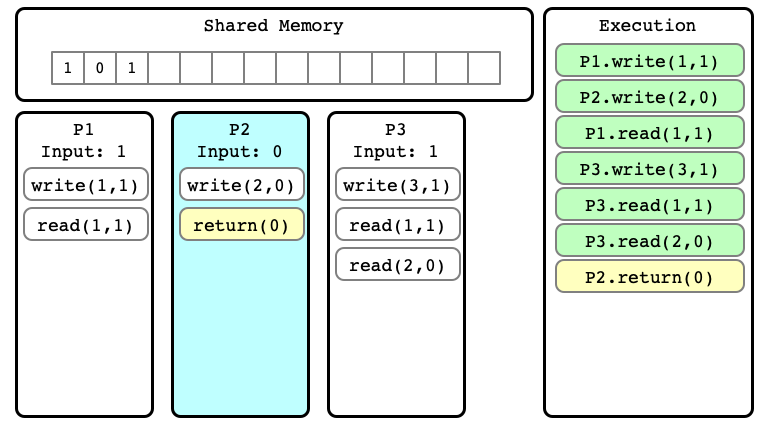$E’$ Step 08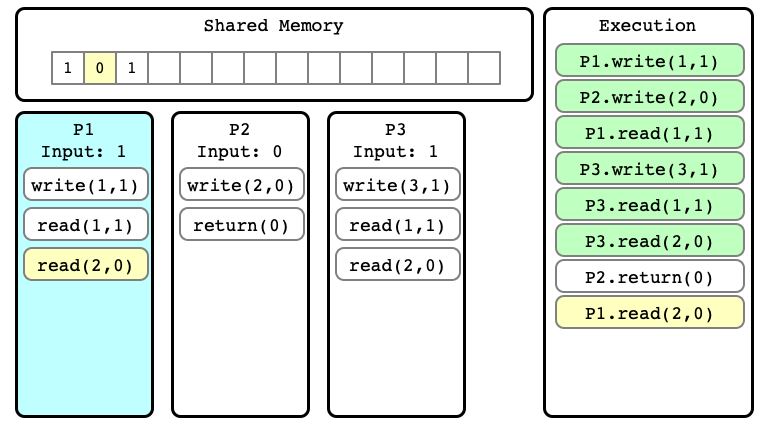$E’$ Step 09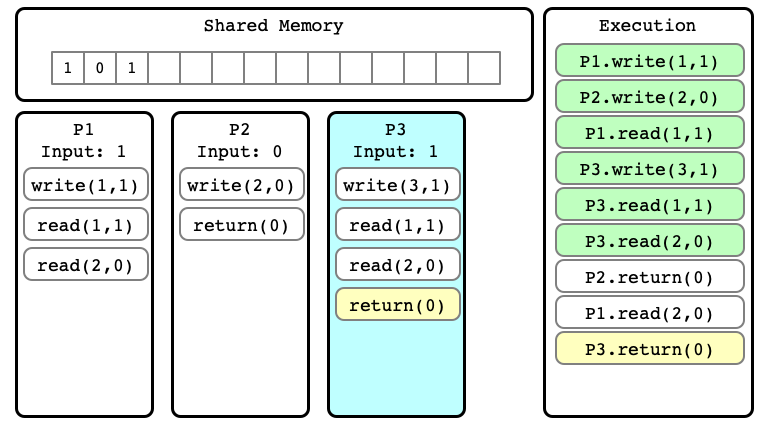$E’$ Step 10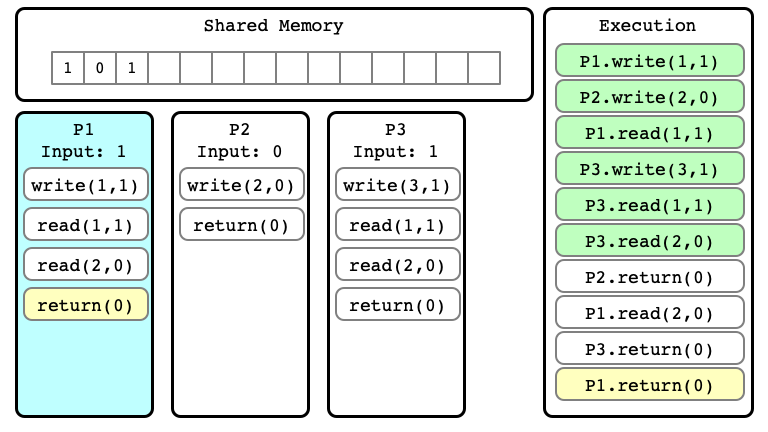Note

We can consider many different extensions of $E$

Extension $E’$ of $E$Alternate extension $E’’$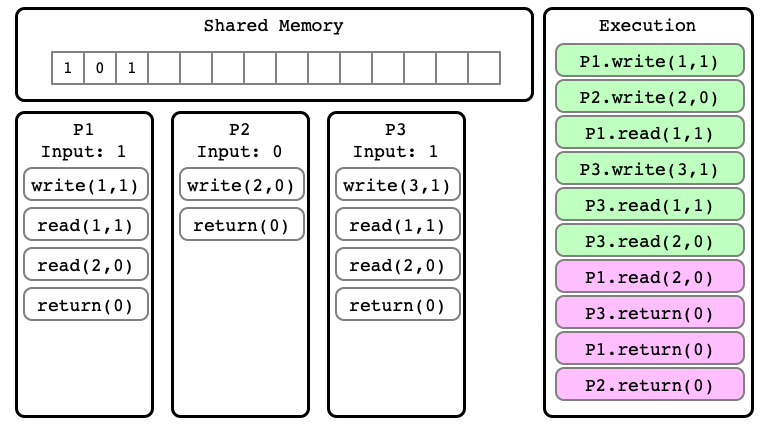Indistinguishable Executions

• $E$ and $E’$ are executions
• they are indistinguishable at process $P_i$ if in $E$ and $E’$:
1. $P_i$ has same input
2. sequence of read/write operations performed by $P_i$ are same
3. the sequence of values read and written by $P_i$ are the same

$E’$ for P1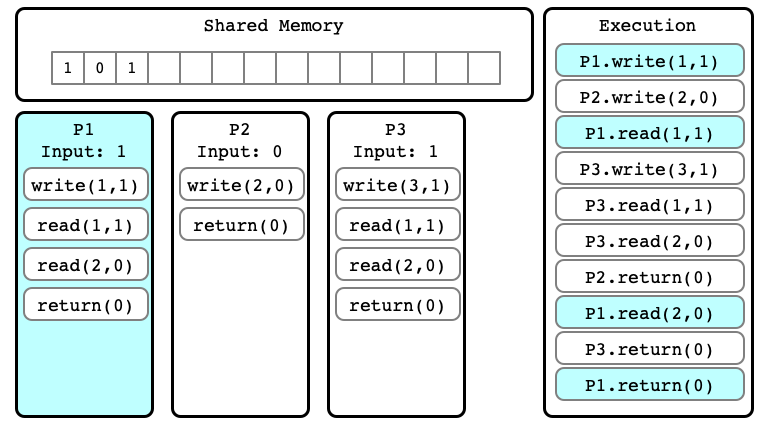$E’’$ for P1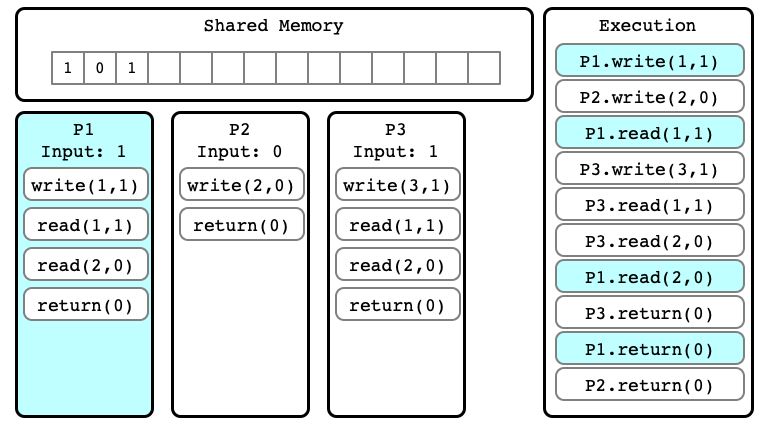First Important Observation

Lemma 1. If executions $E$ and $E’$ are indistinguishable to process $P_i$ then:

1. If $P_i$ has not yet terminated, then $P_i$’s next step will be the same in any extension
2. If $P_i$ has terminated, then $P_i$’s output is the same in $E$ and $E’$

Properties of Consensus Protocols

Main argument for FLP:

1. Describe properties that any hypothetical consensus protocol must have
• bivalent executions
• critical executions
2. Use these properties to show that with only read/write registers there are indistinguishable executions that must give different outputs

Bivalent Executions

• Consider a (hypothetical) wait-free consensus protocol $A$
• Let $E$ be an execution of $A$

We say that $E$ is…

1. $0$-valent if in every extension of $E$, all processes output $0$
2. $1$-valent if in every extension of $E$, all processes output $1$
3. univalent if it is $0$- or $1$-valent
4. bivalent if there exist
• an extension $E’$ of $E$ in which all processes output $0$
• an extension $E’’$ of $E$ in which all processes output $1$

Second Important Observation

Lemma 2. Suppose $A$ solves consensus. Then there is a bivalent initial state.

• Here an initial state is an execution in which no process has yet taken a step
• the execution consists of only inputs for each process

Proof of Lemma 2

Must show: there is a bivalent initial state

Argument:

• by contradiction: suppose no bivalent initial state
• consider sequence of initial states
• show some are $0$-valent, some are $1$-valent
• show that some must be bivalent

$E_1$ is $0$-valent (Why?)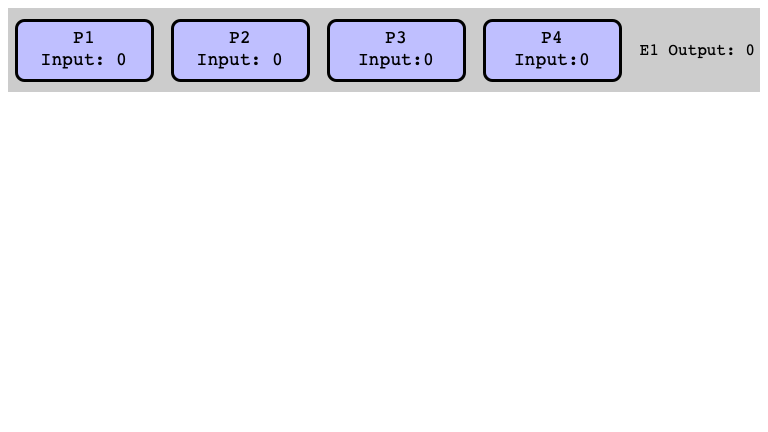$E_5$ is $1$-valent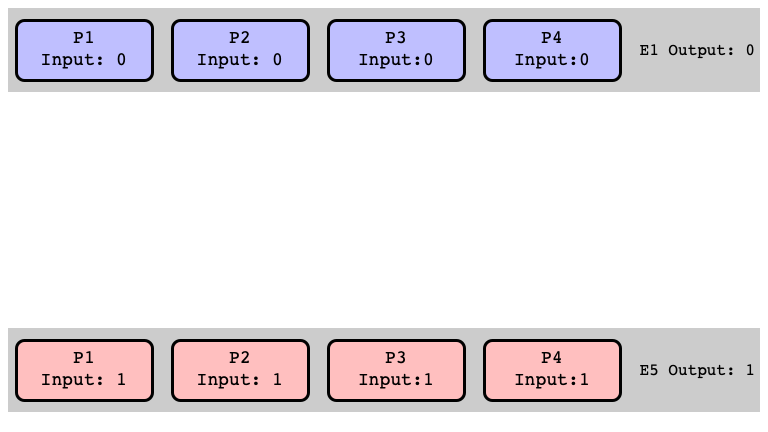More Initial States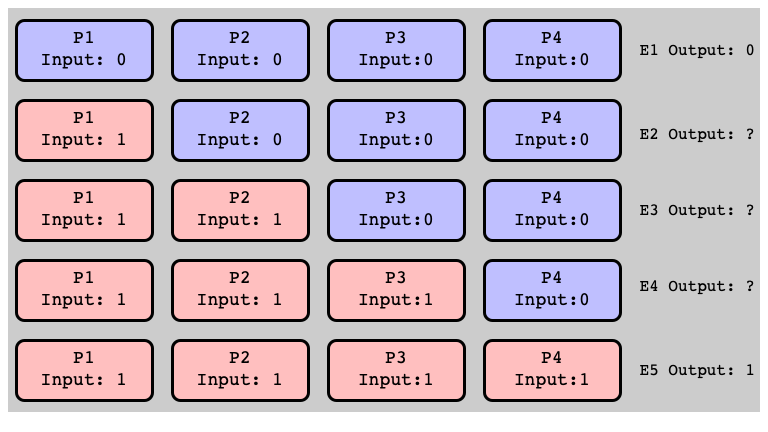Assume: All Univalent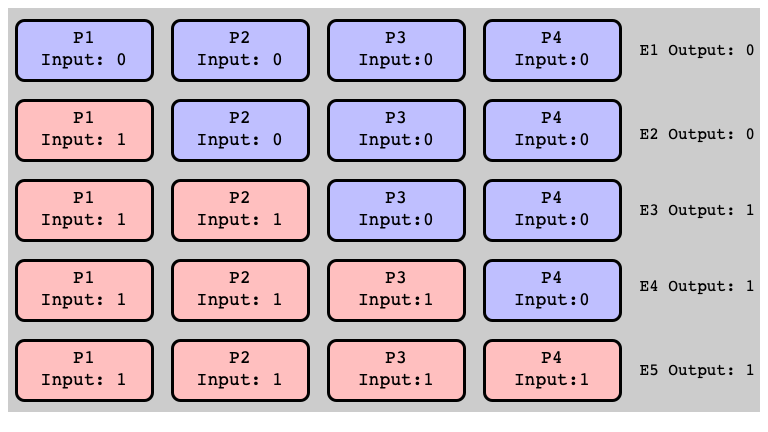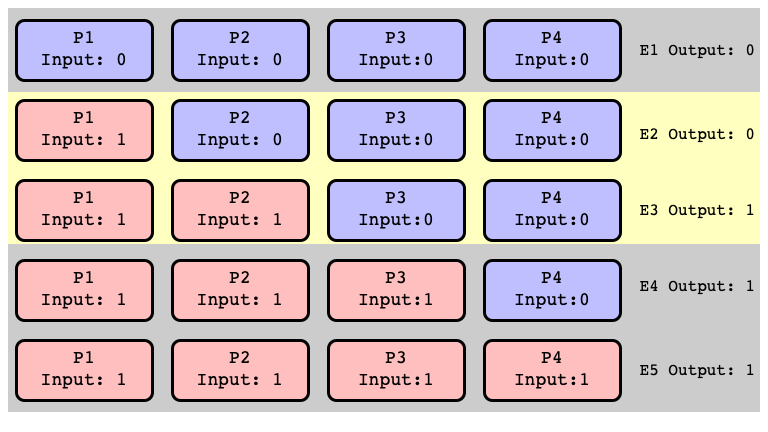All Extensions of $E_2$ Return $0$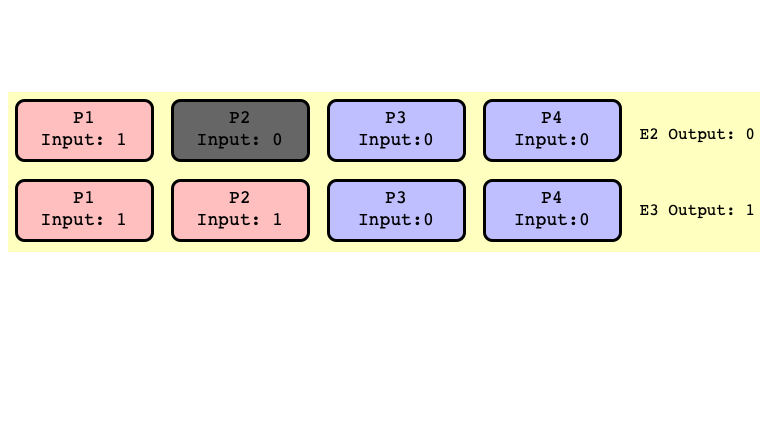All Extensions of $E_3$ Return $1$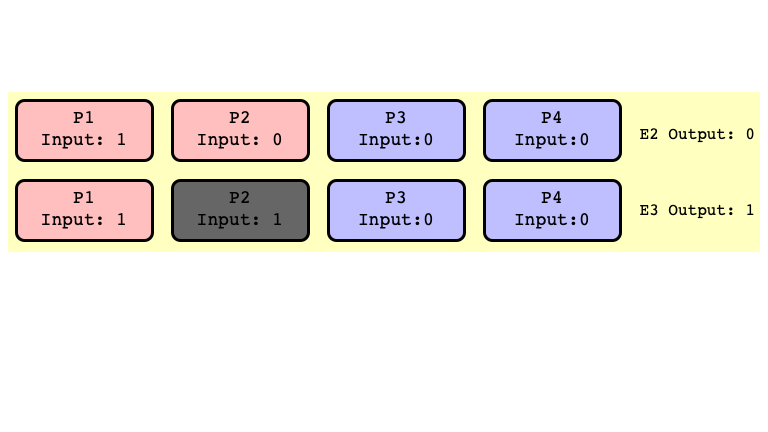$E_2’$ and $E_3’$ Indistinguishable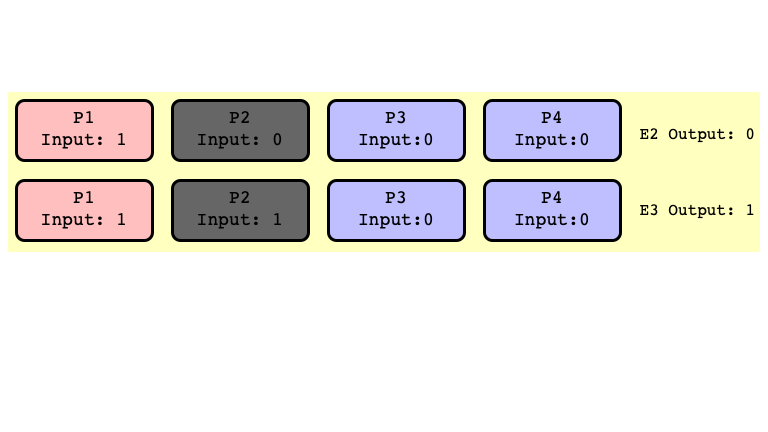$E_2$ and $E_3$ Bivalent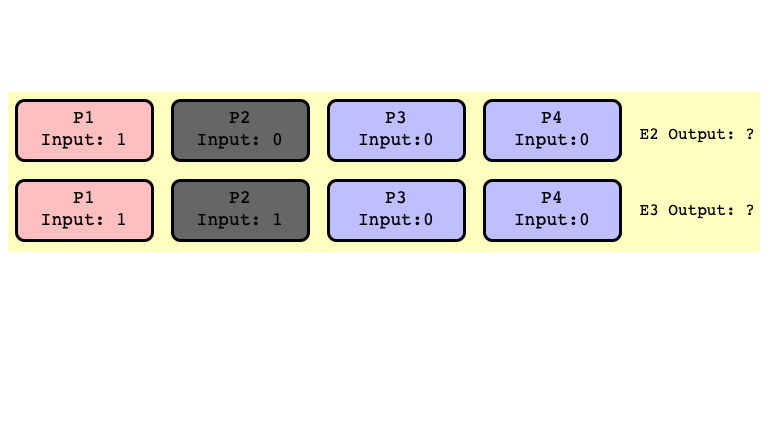Note

Don’t need to assume $P_2$ crashes

• just assume first step of $P_2$ is scheduled after some other thread outputs
• this is possible because we assume $A$ is wait-free
• some process guaranteed to terminate even if one is not scheduled

Mere possibility of a crash together with wait-free assumption implies existence a bivalent initial state

• same holds if we require only termination with one fault

Critical Executions

An execution $E$ is critical if:

1. $E$ is bivalent
2. Extending $E$ by any single step of any process results in a univalent execution

Important Obvservation 3

Lemma 3. Every consensus protocol has a critical execution.

Proof of Lemma 3

Consider a bivalent initial state $E_0$

• Such a state exists by Lemma 2
• If $E_0$ is critical, we’re done
• Otherwise form $E_0, E_1, E_2, \ldots$ where
1. each $E_{i+1}$ extends $E_i$ by single step
2. each $E_i$ is bivalent
• By wait-freedom, the sequence must be finite
• So it has a final $E$ where every extension is univalent
• $E$ is critical!

Properties of Consensus

Lemma 2. Every consensus protocol has a bivalent initial state.

Lemma 3. Every consensus protocol has a citical execution $E$.

So far: Have not used any properties of atomic read/write registers

• These properties hold for all consensus protocols
• even if other atomic operations are supported